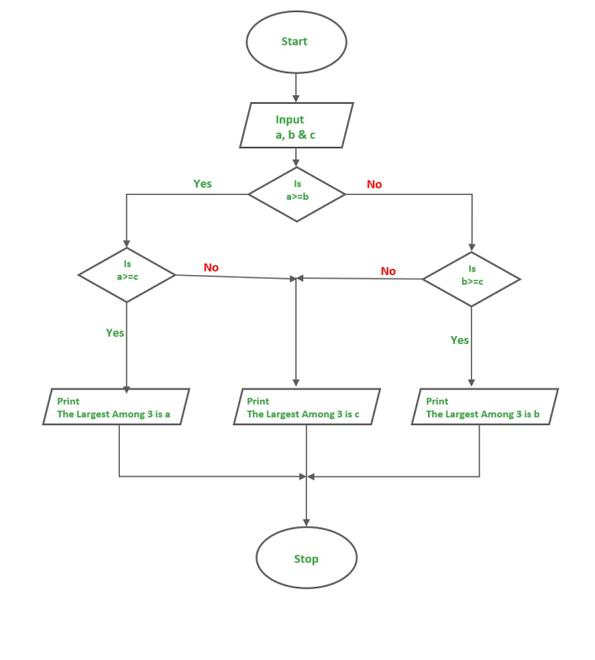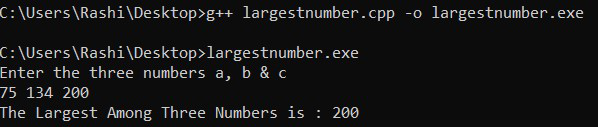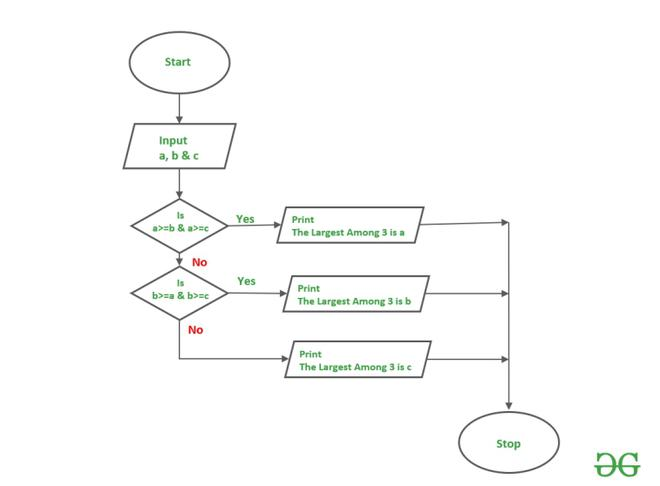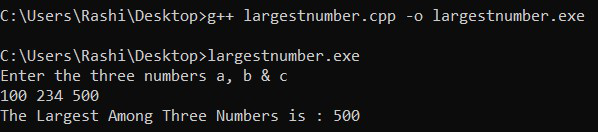# C++ Program to Find Largest Among Three Numbers

• Last Updated : 19 Jul, 2022

Here, we will see how to find the largest among three numbers using a C++ program. Below are the examples:

Input: a = 1, b = 2, c = 45
Output: The Largest Among 3 is 45

Input: a = 75, b = 134, c = 9
Output: The Largest Among 3 is 134

There are 3 ways to find the largest among the three numbers in C++:

1. Using If-else Statement.
2. Using Logical Operators.
3. Using Ternary Operator.

Let’s start discussing each of these methods in detail.

## 1. Using If-else Statement

The following algorithm will be used here:

Algorithm:

1. Start.
2. Input a, b and c.
3. Check the condition a>=b
4. If step 3 is True go to step 5 else go to step 9.
5. Check the condition a>=c.
6. If step 5 is True go to step 7 else go to step 8.
7. Print “The Largest Among 3 is: a and go to step 13
8. Print “The Largest Among 3 is: c and go to step 13.
9. Check the condition b>=c.
10. If step 9 is True go to step 11 else go to step 12.
11. Print “The Largest Among 3 is: b and go to step 13.
12. Print “The Largest Among 3 is: c and go to step 13.
13. Stop.

Flowchart:Below is the C++ program to find the largest among three numbers using the if-else statement:

## C++

 `// C++ Program to Find Largest Among``// Three Numbers Using if-else``// Statement``#include``using` `namespace` `std;` `// Driver code``int` `main()``{``  ``int` `a, b, c;``  ``cout << ``"Enter the three numbers a, b & c"` `<<``           ``endl;``  ``cin >> a >> b >> c;``  ` `  ``if``(a >= b)``  ``{``    ``if``(a >= c)``    ``{``      ``cout << ``"The Largest Among Three Numbers is : "` `<<``               ``a << endl;``    ``}``    ``else``    ``{``      ``cout << ``"The Largest Among Three Numbers is : "` `<<``               ``c << endl;``    ``}``  ``}``  ``else``  ``{``    ``if``(b >= c)``    ``{``      ``cout << ``"The Largest Among Three Numbers is : "` `<<``               ``b << endl;``    ``}``    ``else``    ``{``      ``cout << ``"The Largest Among Three Numbers is : "` `<<``               ``c << endl;``    ``}``  ``}``  ``return` `0;``}`

Output:Time Complexity: O(1)
Auxiliary Space: O(1)

## 2. Using Logical Operator

Below is the flowchart for finding the largest number using the logical operator:Below is the C++ program to find the largest among three numbers using the logical operator:

## C++

 `// C++ program to find largest``// among three numbers using``// logical operator``#include``using` `namespace` `std;` `// Driver code``int` `main()``{``    ``int` `a, b, c;``    ``cout << ``"Enter the three numbers a, b & c"` `<<``             ``endl;``    ``cin >> a >> b >> c;` `    ``if``(a >= b && a >= c)``    ``{``        ``cout << ``"The Largest Among Three Numbers is : "` `<<``                 ``a << endl;``    ``}``    ``else` `if``(b >= a && b >= c)``    ``{``        ``cout << ``"The Largest Among Three Numbers is : "` `<<``                 ``b << endl;``    ``}``    ``else``    ``{``        ``cout << ``"The Largest Among Three Numbers is : "` `<<``                 ``c << endl;``    ``}``    ` `    ``return` `0;``}`

Output:Time Complexity: O(1)
Auxiliary Space: O(1)

3. Using Ternary Operator

Below is the C++ program to find the largest among the three numbers using the ternary operator:

## C++

 `// C++ program to find largest``// among three numbers using``// ternary operator``#include``using` `namespace` `std;` `// Driver code``int` `main()``{``    ``int` `a, b, c, ans;``    ``cout << ``"Enter the three numbers a, b & c"` `<<``             ``endl;``    ``cin >> a >> b >> c;` `    ``ans = (a >= b ? (a >= c ? a : c) :``          ``(b >= c ? b : c));``    ``cout << ``"The Largest Among Three Numbers is : "` `<<``             ``ans << endl;``    ``return` `0;``}`

Output:Time Complexity: O(1)
Auxiliary Space: O(1)

My Personal Notes arrow_drop_up